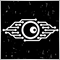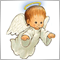Indicator almost ready to go to MQL5 Community. Just 1 bug that is driving me nuts!45558

Just finishing another indicator to share with you on Codebase, but I lost the whole day experimenting, digging, searching, trying to figure out why this behavior is happening.

The indicator is a simple one, based on the famous Didi Needles, but I added a threshold to look for the correct "padding" between 2 of the MAs, so we can filter out supposedly "bad trades". There a re a couple of user inputs, and one of them is the Smoothing of the MAs.

All is working perfectly with ONE bizarre exception. When I select the smoothing "Exponential" the indicator goes crazy! Mixing all data buffers. I checedk every line of code and cannot say why just on the Exponential mode things goes bonkers...

Video:

Could someone take a look and tell me what am I missing?

Thanks a lot in advance!

;)

//+------------------------------------------------------------------+
//| Indicator Settings                                               |
//+------------------------------------------------------------------+
#property indicator_chart_window
#property indicator_buffers 6
#property indicator_plots   6

#property indicator_type1   DRAW_ARROW
#property indicator_color1  clrDodgerBlue
#property indicator_style1  STYLE_SOLID
#property indicator_width1  1

#property indicator_label2  "Sell"
#property indicator_type2   DRAW_ARROW
#property indicator_color2  clrRed
#property indicator_style2  STYLE_SOLID
#property indicator_width2  1

#property indicator_label3  "Fast MA"
#property indicator_type3   DRAW_LINE
#property indicator_color3  clrRed
#property indicator_style3  STYLE_SOLID
#property indicator_width3  2

#property indicator_label4  "Medium MA"
#property indicator_type4   DRAW_LINE
#property indicator_color4  clrYellow
#property indicator_style4  STYLE_SOLID
#property indicator_width4  2

#property indicator_label5  "Slow MA"
#property indicator_type5   DRAW_LINE
#property indicator_color5  clrBlue
#property indicator_style5  STYLE_SOLID
#property indicator_width5  2

#property indicator_label6  "Diff Fast/Slow (%)"
#property indicator_type6   DRAW_NONE

//+------------------------------------------------------------------+
//| Inputs from User Interface                                       |
//+------------------------------------------------------------------+
input int                inpFastPeriod   = 3;              // Fast MA Period
input int                inpMediumPeriod = 8;              // Medium MA Period
input int                inpSlowPeriod   = 20;             // Slow MA Period
input ENUM_MA_METHOD     inpMethod       = MODE_EMA;       // Smoothing Method
input ENUM_APPLIED_PRICE inpAppliedPrice = PRICE_CLOSE;    // MA Price used
input double             inpThreshold    = 0.00;           // Threshold to Filter Entries

//+------------------------------------------------------------------+
//| Global Variables                                                 |
//+------------------------------------------------------------------+
int    bufferBuy_ID=0;    // put names on buffers!
int    bufferSell_ID=1;   //
int    bufferFast_ID=2;   //
int    bufferMedium_ID=3; //
int    bufferSlow_ID=4;   //
int    bufferDiff_ID=5;   //

double bufferBuy[];       // Data/Plot buffers
double bufferSell[];      //
double bufferFast[];      //
double bufferMedium[];    //
double bufferSlow[];      //
double bufferDiff[];      //

int hFast;                // MAs indicators handles
int hMedium;              //
int hSlow;                //

int barsCalculated=0;     // we will keep the number of values in the Moving Average indicator

//+------------------------------------------------------------------+
//| Custom indicator initialization function                         |
//+------------------------------------------------------------------+
int OnInit()   {
int plotStart;

// setting the buffers for indicators and BUY/SELL signals...
SetIndexBuffer( bufferSell_ID,   bufferSell,   INDICATOR_DATA );
SetIndexBuffer( bufferFast_ID,   bufferFast,   INDICATOR_DATA );
SetIndexBuffer( bufferMedium_ID, bufferMedium, INDICATOR_DATA );
SetIndexBuffer( bufferSlow_ID,   bufferSlow,   INDICATOR_DATA );
SetIndexBuffer( bufferDiff_ID,   bufferDiff,   INDICATOR_DATA );

// prepare the arrows for the entry signals...
PlotIndexSetInteger(bufferSell_ID,PLOT_ARROW,234);

PlotIndexSetInteger(bufferSell_ID,PLOT_ARROW_SHIFT,-10);

// sets the starting point for all 3 MAs plotting buffers...
plotStart = MathMax( inpFastPeriod, MathMax(inpMediumPeriod, inpSlowPeriod) );
PlotIndexSetInteger( bufferFast_ID, PLOT_DRAW_BEGIN, plotStart );
PlotIndexSetInteger( bufferMedium_ID, PLOT_DRAW_BEGIN, plotStart );
PlotIndexSetInteger( bufferSlow_ID, PLOT_DRAW_BEGIN, plotStart );

// opens the indicators...
hFast   = iMA(Symbol(), Period(), inpFastPeriod,   0, inpMethod, inpAppliedPrice);
hMedium = iMA(Symbol(), Period(), inpMediumPeriod, 0, inpMethod, inpAppliedPrice);
hSlow   = iMA(Symbol(), Period(), inpSlowPeriod,   0, inpMethod, inpAppliedPrice);

if(hFast==INVALID_HANDLE || hMedium==INVALID_HANDLE || hSlow==INVALID_HANDLE) { // is there anything wrong?
Print("Error starting MA indicators!");
return(INIT_FAILED);
}

return(INIT_SUCCEEDED);
}

//+------------------------------------------------------------------+
//| Custom indicator iteration function                              |
//+------------------------------------------------------------------+
int OnCalculate(const int rates_total,
const int prev_calculated,
const datetime &time[],
const double &open[],
const double &high[],
const double &low[],
const double &close[],
const long &tick_volume[],
const long &volume[],
const int &spread[])   {

int  values_to_copy;                  //number of values copied from the indicator
int  i;

// determine the number of values already calculated in the indicator
int calculated=BarsCalculated(hFast);
if (calculated<=0) {
PrintFormat("BarsCalculated() returned %d, error code %d",calculated,GetLastError());
return(0);
}

// if it is the first start of calculation of the indicator or if the number of values in the indicator changed
// or if it is necessary to calculated the indicator for two or more bars (it means something has changed in the price history)
if (prev_calculated == 0 || calculated != barsCalculated || rates_total > prev_calculated+1) {
// if the iMABuffer array is greater than the number of values in the indicator for symbol/period, then we don't copy everything
// otherwise, we copy less than the size of indicator buffers
if (calculated>rates_total) {
values_to_copy = rates_total;
} else {
values_to_copy = calculated;
}
} else {
// it means that it's not the first time of the indicator calculation, and since the last call of OnCalculate()
// for calculation not more than one bar is added
values_to_copy = (rates_total-prev_calculated) + 1;
}

// fill the MA buffers arrays with values of the Moving Average indicators...
// if FillArrayFromBuffer returns false, it means the information is nor ready yet, quit operation
if (!FillArrayFromBuffer( hFast,   0, values_to_copy, bufferFast ) )    {  return(0);  }
if (!FillArrayFromBuffer( hMedium, 0, values_to_copy, bufferMedium ) )  {  return(0);  }
if (!FillArrayFromBuffer( hSlow,   0, values_to_copy, bufferSlow ) )    {  return(0);  }

// memorize the number of values in the Moving Average indicator
barsCalculated=calculated;

// processes the BUY and SELL signals
for (i=MathMax(1,values_to_copy-1); i<rates_total; i++)  {

bufferDiff[i] = MathAbs( (bufferFast[i]/bufferSlow[i]-1)*100 );    // calculates the diff % between the Fast and Slow MAs

if ( (bufferFast[i]>bufferFast[i-1] && bufferMedium[i]>bufferMedium[i-1] && bufferSlow[i]>bufferSlow[i-1]) &&   // upward aligned MAs
(bufferFast[i]>bufferMedium[i] && bufferFast[i]>bufferSlow[i] && bufferMedium[i]>bufferSlow[i]) &&         // MAs aligned for a BUY
(bufferFast[i]>=open[i] && bufferFast[i]<=close[i] && bufferMedium[i]>=open[i] && bufferMedium[i]<=close[i] && bufferSlow[i]>=open[i] && bufferSlow[i]<=close[i]) &&  // All MAs inside the candle body
(bufferDiff[i] >= inpThreshold)   // and only if the diff between MAs Fast/Slow is greater than the threshold
)  {
bufferSell[i] = EMPTY_VALUE;  //do not print an arrow

// SELL Signal
} else if ( (bufferFast[i]<bufferFast[i-1] && bufferMedium[i]<bufferMedium[i-1] && bufferSlow[i]<bufferSlow[i-1]) &&   // downward aligned MAs
(bufferFast[i]<bufferMedium[i] && bufferFast[i]<bufferSlow[i] && bufferMedium[i]<bufferSlow[i]) &&         // MAs aligned for a SELL
(bufferFast[i]<=open[i] && bufferFast[i]>=close[i] && bufferMedium[i]<=open[i] && bufferMedium[i]>=close[i] && bufferSlow[i]<=open[i] && bufferSlow[i]>=close[i]) &&  // All MAs inside the candle body
(bufferDiff[i] >= inpThreshold)   // and only if the diff between MAs Fast/Slow is greater than the threshold
) {
bufferSell[i] = high[i];
bufferBuy[i]  = EMPTY_VALUE;  //do not print an arrow

} else {
bufferBuy[i] = bufferSell[i] = EMPTY_VALUE;  //do not print an arrow
}

}

// return the prev_calculated value for the next call
return(rates_total);
}

//+------------------------------------------------------------------+
//| Filling indicator buffers from the MA indicator                  |
//+------------------------------------------------------------------+
bool FillArrayFromBuffer( int indicatorHandle,          // handle of the instantiated Indicator
int indicatorInternalBuffer,  // internal buffer # of the Indicator (iMAs == 0, just one!)
int itemsQty,                 // number of values to copy to buffer
double &bufferToCopyInto[],   // buffer to hold a copy of indicator's data
) {

ResetLastError();   // reset last error code

// fill a part of the requested buffer array with values from the indicator buffer that has a 0 index
if (CopyBuffer(indicatorHandle, indicatorInternalBuffer, 0, itemsQty, bufferToCopyInto) < 0) {  // if the copying fails, tell the error code
Print( "Failed to copy data from the Indicator. Error Code:", GetLastError());
return(false);  // quit with zero result - it means that the indicator is considered as not calculated
}
return(true);  // everything is fine
}

//+------------------------------------------------------------------+
//| Custom indicator deinitialization function                       |
//+------------------------------------------------------------------+
void OnDeinit(const int reason) {
IndicatorRelease(hFast);
IndicatorRelease(hMedium);
IndicatorRelease(hSlow);
}
//+------------------------------------------------------------------+
Files:2856

Minions Labs:

Could someone take a look and tell me what am I missing?

Thanks a lot in advance!

;)

Apparently you try division by zero.

bufferDiff[i] = MathAbs( (bufferFast[i]/bufferSlow[i]-1)*100 );